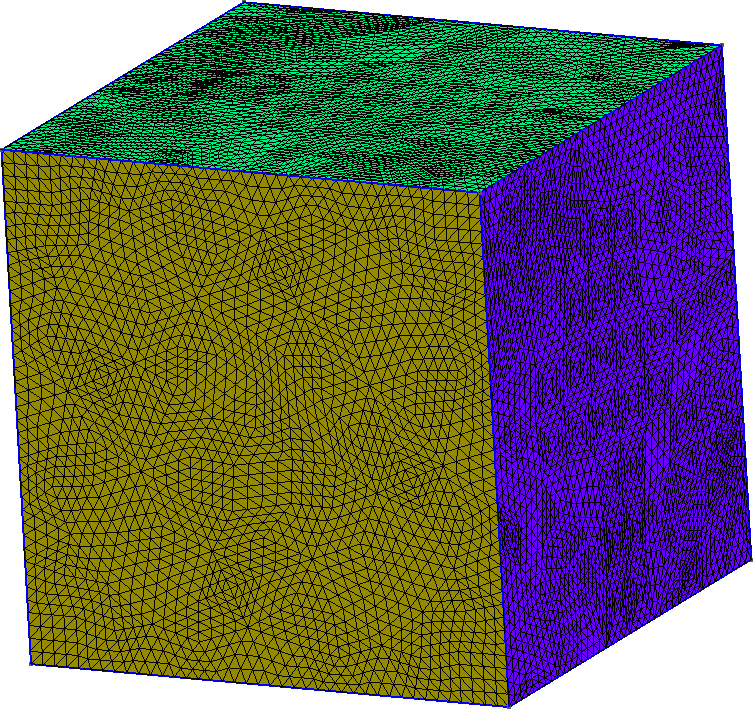# More geometries

Only few of the possibilities are shown here. Please have a look at the official documentation for every other operations.

## Circular curve

Open a new file, `circle.geo`, and insert the following code:

``````DefineConstant[
R = {1, Min 0.5, Max 10, Step 0.1, Name "R"},
h = {0.1, Min 0.01, Max 10, Step 0.01, Name "h"}
];
xc = 0;
yc = 0;
Point(1) = {xc, yc, 0, h};
Point(2) = {xc + R, yc, 0, h};
Point(3) = {xc, yc + R, 0, h};
Point(4) = {xc - R, yc, 0, h};
Point(5) = {xc, yc - R, 0, h};
Circle(1) = {2,1,3};
Circle(2) = {3,1,4};
Circle(3) = {4,1,5};
Circle(4) = {5,1,2};
Curve Loop(1) = {1,2,3,4};   // Boundary
Plane Surface(1) = {1};     // Surface
Physical Surface(1) = {1};  // Physical Tag
``````

GMSH should display a circle and a menu on the left with 2 parameters that can be modified on runtime :Parameters can be modified on the left menu

What is defined in `DefineConstant` is explained in the section dedicated to the GUI (Graphical User Interface) but basically, these lines add parameters that can be modified by the user in the GUI.

What is interesting here is the `Circle` commands that draws an arc (with angle less than π). It needs three `Point` to be fully defined (start, center, end):

``````Circle(index) = {PointA, PointCenter, PointB};
``````

Note that drawing a circle needs 3 points at minimum (instead of 4).

`Circle` can not draw arc with angle equal or greater than π.
`Circle` and `Line` share the same index counter: a `Line` and a `Circle` cannot share the same index!

Time to play:

1. Introduce a circle of center point (0,0) and of radius `Rint` that can be modified in the GUI, with minimum value 0.1, maximum value 0.4 and a `Step` of 0.1. You can copy/paste the line where `R` is defined and modify it accordingly.
2. Modify the definition of the `Surface` to make it looks like a donut instead of a disk## `Extrude`: from a square to a cube

Extrusion is a nice way to build 3D geometries from 2D:

``````    h = 1;                     // Characteristic length of a mesh element
Point(1) = {0, 0, 0, h};   // Point construction
Point(2) = {10, 0, 0, h};
Point(3) = {10, 10, 0, h};
Point(4) = {0, 10, 0, h};
Line(1) = {1,2};            //Lines
Line(2) = {2,3};
Line(3) = {3,4};
Line(4) = {4,1};
Curve Loop(1) = {1,2,3,4};   // A Boundary
Plane Surface(1) = {1};     // A Surface

Extrude {0, 0, 10} { Surface{1};}  // Extrusion!
Physical Volume(1) = {1};          // Setting a label to the Volume
``````

The square of width 10 (`{ Surface{1};}`) is extruded (`Extrude`) in the z-direction with length `10` (`{0, 0, 10}`). Applying this code in GMSH gives the following figure:Mesh of the cube